Bio-macro molecules (2011 VCE) 1) The following is a diagram of a section of a protein chain.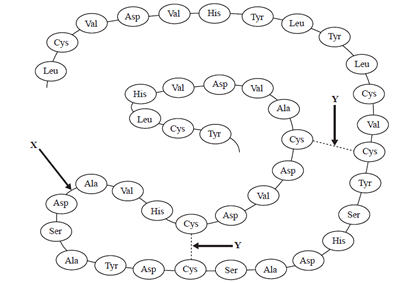The bonds represented by X and Y are: a) amide bond and disulfide bond; b) covalent bond and ionic bond; c) hydrogen bond and ionic bond; d) dipole-dipole bond and covalent bond. Solution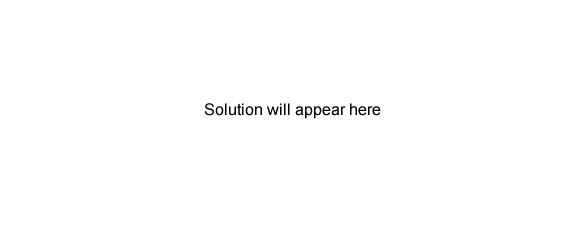2) Below are the structures of a number of important molecules.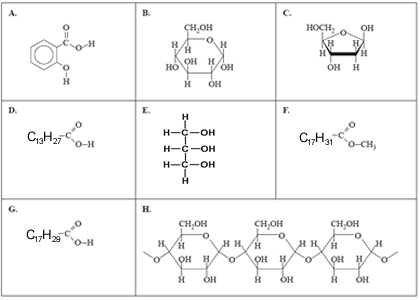In each of the questions select the letter that corresponds to the letter of the molecule above. a) Which molecule is used in the synthesis of acetylsalicylic acid? A B C D E F G H b) Which molecule is the product of a condensation reaction? A B C D E F G H c) Which two molecules are produced when a triglyceride containing no carbon-carbon double bonds undergoes hydrolysis? A B C D E F G H and A B C D E F G H d) Which molecule could be a major component of biodiesel? A B C D E F G H e) Which molecule is one of the components that reacts to form sucrose? A B C D E F G H f) 0.001 mole of which molecule will react exactly with 0.32 grams of bromine? A B C D E F G H Solution Examiner comments to f above.3) a) The letters GATAC represent a part of the base sequence in a strand of DNA. In the boxes provided write the base sequence of the complementary strand of DNA. B C U T G A B C U T G A B C U T G A B C U T G A B C U T G A B C U T G A Solution b) Determine the number of hydrogen bonds between the base pairings in the segment of DNA. Solution c) Four different monomers known as nucleotides combine in a condensation reaction to form a strand of DNA. One such nucleotide is shown below.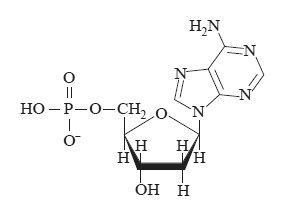Name the reactants that have combined in condensation reactions to form this nucleotide? Solution4) Bradykinin is a peptide that lowers blood pressure.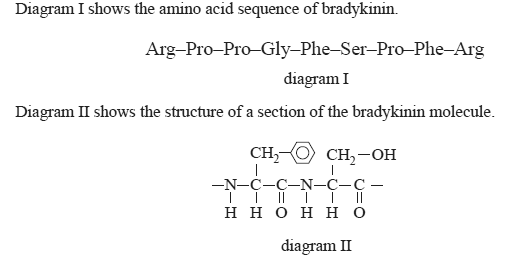a) On diagram I circle the section of bradykinin that is represented in diagram II. Solution b) Peptides can be completely hydrolysed to their component amino acids by treatment with 6M HCl. Identify the two functional groups that are formed as a result of the hydrolysis of the peptide link. Solution c) Draw the chemical structure, showing all bonds, of the amino acid glycine as it would exist in solution at pH=1. Solution5) A starch molecule contains 500 glucose units. If the molar mass of glucose is 180 g/mol, then the molar mass of the starch molecule is a) 8982 g/mol b) 81018 g/mol c) 90000 g/mol d) 98982 g/mol Solution